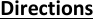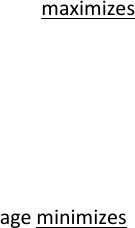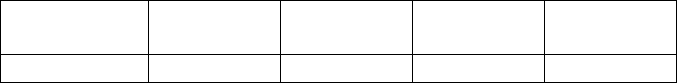# ECO 311 Miami U eco311mt1Fall 1fall17

29 views8 pages
School
Miami University
Department
Economics
Course
ECO 311
ProfessorEco311, First Midterm Exam, Fall 2017
Prof. Bill Even
-1-
Directions
distinguished from your work. If the logic of your work looks correct but there is a math error, you may
follow.
Round all numerical answers to the nearest 100th (e.g. 1.23) unless specifically told otherwise. Unless
indicated otherwise, each question is worth 4 points.
The formula sheet and a table with the standard normal CDF are attached to the last page of the exam.
Unlock document

This preview shows pages 1-3 of the document.
Unlock all 8 pages and 3 million more documents.Eco311, First Midterm Exam, Fall 2017
Prof. Bill Even
-2-
To answer the next 3 questions, consider the following relationship between annual labor earnings
(incwage), age, and age-squared that was estimated using data from the 2015 American Community
Survey.
Incwage = -72000 + 5240**age 51*age2
1. If a worker is 40 years old, use a derivative to calculate the marginal effect of one more year of age on
annual earnings.
In the ACS data, workers range between 16 and 97 years of age.
2. What age maximizes annual earnings? (Your answer must be somewhere in the range of 16 to 97
years of age).
3. What age minimizes annual earnings? (Your answer must be somewhere in the range of 16 to 97
years of age).
4. As an alternative to the linear model above, consider the following log-linear model of annual
earnings:
ln(incwage)=5.4 + .22*age- .002*age2
For a worker who is 40 years old, use a log-approximation to estimate the percent change in earnings
associated with an additional year of age. Be sure to give your answer as a percent (e.g. 20.32%, not
.2032).
Unlock document

This preview shows pages 1-3 of the document.
Unlock all 8 pages and 3 million more documents.Eco311, First Midterm Exam, Fall 2017
Prof. Bill Even
-3-
Consider the alternative to the earlier equation for annual earnings where earnings are allowed to
depend on age and years of education (educ)
Incwage= -27000 + 265*age +5700*educ + 60*(age*educ)
5. Based on this equation for annual earnings, use a first order approximation to estimate the marginal
effect of an additional year of age for a person who is 40 years old with 12 years of school.
6. Based on the above equation, which of the following is true?
a. The marginal effect of age on income is greater for more educated workers.
b. The marginal effect of education on income is greater for older workers
c. Both of the above are true
d. None of the above are true.
To answer the next 3 questions, consider the following data on the number of times a person has been
married drawn from the 2015 American Community Survey. Those who were married more than 3
times are recorded as a 3. You can ignore this issue in answering the questions. I also rounded the
probabilities to the nearest .05 to make calculations easier. Show the basic steps behind your work for
Number of
times married
0
1
2
3
Probability
.30
.55
.10
.05
7. What is the expected number of times that a person has been married?
8. What is the variance of number of times married?
9. What is [( − ) 3 ] where X is number of times married and is the expected value of X?
Unlock document

This preview shows pages 1-3 of the document.
Unlock all 8 pages and 3 million more documents.